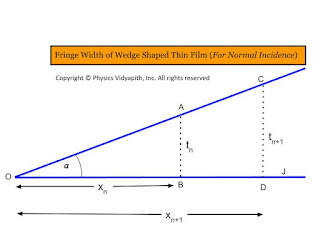### Fringe width of wedge shaped thin film for normal incidence

Derivation of Fringe width of the wedge-shaped thin film:
• The distance between two consecutive bright (or dark) fringes is called the fringe width.

• If the $n^{th}$ bright fringe is formed at a distance $x_{n}$ from the edge of the wedge shaped film where the thickness is $t_{n}$. So the path difference for $x_{n}$ bright fringe:

• $(2n-1) \frac{\lambda}{2}= 2 \mu \: t_{n} \: cos(\alpha+r) \qquad(1)$

For Normal IncidenceThe incident Angle $i=0$The refracted Angle $r=0$

then from equation $(1)$

$(2n-1) \frac{\lambda}{2}= 2 \mu \: t_{n} \: cos\alpha \qquad(2)$Fringe width of wedge-shaped thin film for normal incidence

From the figure, In $\Delta OAB$

$tan \alpha = \frac{t_{n}}{x_{n}}$

$t_{n}=x_{n} \: tan \alpha \qquad(3)$

Now put the value of $t_{n}$ in equation $(2)$

$(2n-1) \frac{\lambda}{2}= 2 \mu \: x_{n} \: tan \alpha \: cos\alpha$

$(2n-1) \frac{\lambda}{2}= 2 \mu \: x_{n}\: \frac{sin \alpha}{cos\alpha} \: cos\alpha$

$(2n-1) \frac{\lambda}{2}= 2 \mu \: x_{n} \: sin \alpha \qquad(4)$

Similarly for $(n+1)^{th}$ bright fringe i.e put the $(n+1)$ in place of $n$ in above equation then we get

$\left\{ 2(n+1)-1 \right\} \frac{\lambda}{2}= 2 \mu \: x_{n+1} \: sin \alpha$

$(2n+1) \frac{\lambda}{2}= 2 \mu \: x_{n+1} \: sin \alpha \qquad(5)$

Subtract the equation $(5)$ and equation$(4)$

$2\mu \: (x_{n+1}-x_{n})\: sin \alpha =\lambda$

$2\mu \beta \: sin \alpha =\lambda$

Where $\beta=$ fringe Width$(x_{n+1}-x_{n})$

 $\beta=\frac{\lambda}{2\mu\: sin \alpha}$

If $\alpha$ is very small so $sin \alpha \approx \alpha$

 $\beta=\frac{\lambda}{2\mu\: \alpha}$